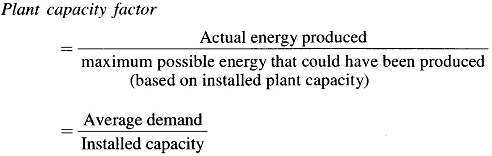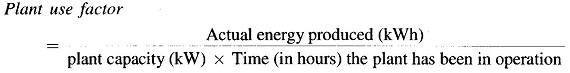Home » Introduction to Modern Power System » Diversity Factor in Power System

## Diversity Factor in Power System:

Diversity Factor in Power System is defined as the sum of individual maximum demands on the consumers, divided by the maximum load on the system. This factor gives the time diversification of the load and is used to decide the installation of sufficient generating and transmission plant. If all the demands came at the same time, i.e. unity diversity factor, the total installed capacity required would be much more. Luckily, the factor is much higher than unity, especially for domestic loads.

A high Diversity Factor in Power System could be obtained by:

1. Giving incentives to farmers and/or some industries to use electricity in the night or light load periods.
2. Using day-light saving as in many other countries.
3. Staggering the office timings.
4. Having different time zones in the country like USA, Australia, etc.
5. Having two-part tariff in which consumer has to pay an amount dependent on the maximum demand he makes, plus a charge for each unit of energy consumed. Sometimes consumer is charged on the basis of kVA demand instead of kW to penalize loads of low power factor.

Two other factors used frequently are:### Tariffs:

The cost of electric power is normally given by the expression (a + b x kW + c x kWh) per annum, where a is a fixed charge for the utility, independent of the power output; b depends on the maximum demand on the system and hence on the interest and depreciation on the installed power station; and c depends on the units produced and therefore on the fuel charges and the wages of the station staff.

Tariff structures may be such as to influence the load curve and to improve the load factor.

Tariff should consider the pf (power factor) of the load of the consumer. If it is low, it takes more current for the same kWs and hence T and D (transmission and distribution) losses are correspondingly increased. The power station has to install either pf correcting (improvement) devices such as synchronous capacitors, SVC (Static Var Compensator) or voltage regulating equipment to maintain the voltages within allowed limits and thus total cost increases. One of the following alternatives may be used to avoid low pf:

• to charge the consumers based on kVA rather than kW.
• a pf penalty clause may be imposed on the consumer.
• the consumer may be asked to use shunt capacitors for improving the power factor of his installations